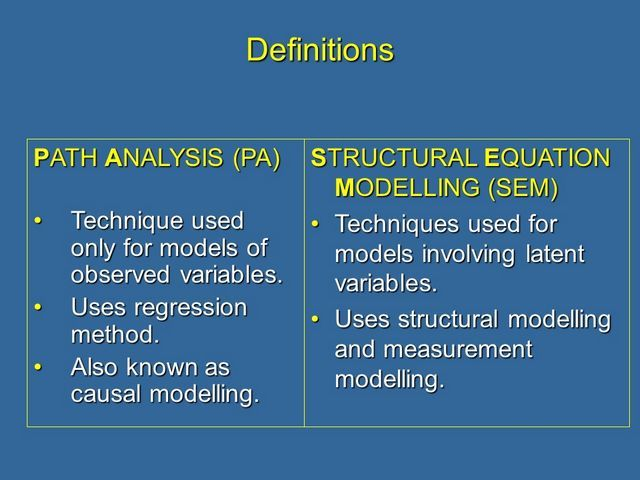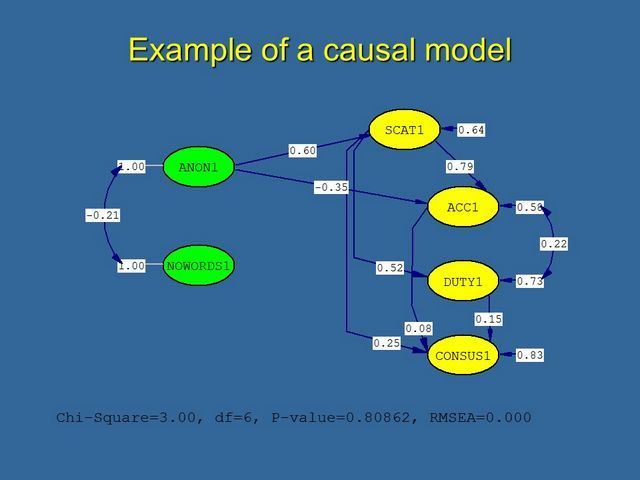# 1. What is Path Analysis?

Path analysis and structural equation modeling are techniques to assess the direct causal contribution of one variable to another in a non experimental situation. They are therefore particularly useful in field studies, and have become increasingly popular as modern psychology draws from real problems and non-laboratory research methods. However, as I’ll show, path analysis can be very useful in laboratory experiments as well, where it can be used to determine the psychological processes mediating the effects of independent variables on behaviour. Structural equation modelling is basically a more complex form of path analysis. So let’s start with some basic definitions.

## DefinitionsFirst, some basic definitions of terms.

Path analysis has traditionally been known as causal modelling. It is used on data consisting of sets of observed variables. The technique uses the multiple regression method.

Structural equation modelling is basically a more complex form of path analysis. It is used where models involve latent variables. Latent variables are hypothetical constructs that relate to more than one observed variable. (I shall explain this later).

Full structural equation modelling involves structural modelling AND also measurement modelling. It can be regarded as combining the techniques of path analysis and factor analysis in one step to produce estimates of the causal relations among a set of variables.

## Example of a Causal Model

Below is an example of a causal model I made earlier. This actually models the results of an actual experiment carried out by one of my postgraduates. As you can see, causal models can be quite complex. However, they can be produced quite simply these days thanks to the development of specific programs. You may have come across the names: EQS, AMOS and LISREL are the most famous. Despite the range of programs available they all do the same kinds of analyses in pretty much the same ways. You’ll be learning to use AMOS.